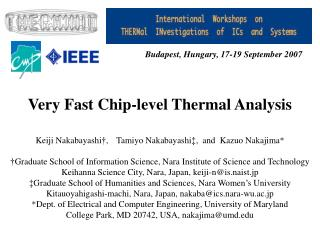DownloadDownload PresentationVery Fast Chip-level Thermal Analysis

# Very Fast Chip-level Thermal Analysis

Download Presentation## Very Fast Chip-level Thermal Analysis

- - - - - - - - - - - - - - - - - - - - - - - - - - - E N D - - - - - - - - - - - - - - - - - - - - - - - - - - -
##### Presentation Transcript

1. Very Fast Chip-level Thermal Analysis Budapest, Hungary, 17-19 September 2007 Keiji Nakabayashi†, Tamiyo Nakabayashi‡, and Kazuo Nakajima* †Graduate School of Information Science, Nara Institute of Science and Technology Keihanna Science City, Nara, Japan, keiji-n@is.naist.jp ‡Graduate School of Humanities and Sciences, Nara Women’s University Kitauoyahigashi-machi, Nara, Japan, nakaba@ics.nara-wu.ac.jp *Dept. of Electrical and Computer Engineering, University of Maryland College Park, MD 20742, USA, nakajima@umd.edu

2. Abstarct We present a new technique of VLSI chip-level thermal analysis. We extend a newly developed method of solving two dimensional Laplace equations to thermal analysis of four adjacent materials on a mother board. We implement our technique in C and compare its performance to that of a commercial CAD tool. Our experimental results show that our program runs 5.8 and 8.9 times faster while keeping smaller residuals by 5 and 1 order of magnitude, respectively.

3. 1. Introduction • Thermal phenomena is very important factor in VLSI and board design in the post-90 nm era. • Thermal analysis is modeling of thermal conduction by a Laplace equation and its solution by finite difference method (FDM). • We developed a new, very fast chip-level thermal analysis technique.

4. 2. Problem • Consider a multi-layer structure, where four layers of materials p,q,r,and s of thermal conductivities kp, kq, kr, and ks, respectively, are stacked together. • Heat travels through a heat transfer pass consisting of the chip die, the adhesive, the heat spreader, and the heat sink, and goes out to the ambient air. • Our problem is to find temperature distribution through two dimensional steady-state thermal conduction analysis.

5. 3. Method • Recently, a new efficient direct method, called Symbolic Partial Solution Method (S-PSM) was developed in the area of computational fluid dynamics. • S-PSM-based solution process goes through many levels of repeated operations of decomposition and merging. • We extend this S-PSM-based Laplace equation solver to a multi-layer structure.

6. h X Tleft=20(℃) (Reference) Tright=20(℃) (Reference) 2048um Tamb=55(℃) (Ambient temperature) np+2 128um Heak sink kp= 260 [W/(m・K)] nq+2 128um Heak spreader kq= 230 [W/(m・K)] nr+2 g 64um Adhesive kr= 16 [W/(m・K)] 256um ns+2 Chip die ks= 100 [W/(m・K)] Y (0,0) Tj=120(℃) （Heat source: Si bulk temperature) Cell h : number of Y-axis grid points g : number of X-axis grid points where g = (np+2)+(nq+2)+(nr+2)+(ns+2) Cell size : = = 2048(um) / h Boundary Value Problem (BVP)

7. The arrangement of interior grid and boundary points for the four material domains. kp kq kr ks

8. Laplace Equation and Finite Difference Method for each material u (=p, q, r, s): Finite Difference Method Final Solution for each material :

9. thermal conductivity of the boundary between adjacent materials From the viewpoint of material q, the following difference equation holds at its boundary with material p (first order approximation) : Similarly, at its boundary with material r,

10. For materials p, q, r, swithkqr Final solutions: Eq. (3-35) ↑ Final equations: Eq. (3-33) For materials p, qwithkpq For materials r, s withkrs {q5, r0} Solutions: Eq. (3-31) ↑ Equations: Eq. (3-28) Solutions: Eq. (3-32) ↑ Equations: Eq. (3-29) Back substitution {p0, p5, q0} {r5, s0, s5} Solutions: Eq. (3-24) ↑ Equations: Eq. (3-20) Solutions: Eq. (3-25) ↑ Equations: Eq. (3-21) Solutions: Eq. (3-26) ↑ Equations: Eq. (3-22) Solutions: Eq. (3-27) ↑ Equations: Eq. (3-23) For material p withkp For material qwithkq For material r withkr For material s withks {p1, p4} {q1, q4} {r1, r4} {s1, s4} Decompose Equations Eq. (3-19) into 4 subsystems { } : Variables with known values. : Merging operation for adjacent partial solutions.

11. System Decomposition and Partial Solutions • for Each Subsystem/Material (3-20) (3-21) Decomposition (3-22) (3-23)

12. (3-24) (3-25) Partial solution (3-26) (3-27)

13. (3-28) Merge (3-29) where

14. (3-31) Partial solution (3-32) Merge (3-33) Final solution (3-35)

15. Temperature distributions of steady-state heat conduction for four layers of materials : our program vs. commercial tool Raphael Our Program Solver: S-PSM Raphael  Solver : Iteration method

16. 4. Results and Discussion • Table I shows the CPU times required and the residuals produced by our program and Raphael. • The results demonstrate that for the largest grid, our program ran 5.8 and 8.9 times faster while keeping smaller residuals by 5 and 1 order of magnitudes, than Raphael 

17. 5. Conclusions • We have proposed a new technique of solving two dimensional Laplace equations to thermal analysis for multi-layer VLSI chips. • Our program is superior to a commercial CAD tool, Raphael (iteration method) . • Further Work : extension to Poisson equation (heat generation), three dimensional, transient heat conduction analysis, and the case of complex shapes and boundary conditions of materials.